Courses

# Ex 4.3 NCERT Solutions - Linear Equations in Two Variables Class 9 Notes | EduRev

## Mathematics (Maths) Class 9

Created by: Full Circle

## Class 9 : Ex 4.3 NCERT Solutions - Linear Equations in Two Variables Class 9 Notes | EduRev

The document Ex 4.3 NCERT Solutions - Linear Equations in Two Variables Class 9 Notes | EduRev is a part of the Class 9 Course Mathematics (Maths) Class 9.
All you need of Class 9 at this link: Class 9

Question 1: Draw the graph of each of the following linear equations in two variables: (i) x + y = 4 (ii) x – y = 2 (iii) y = 3x (iv) 3 = 2x + y
Solution: (i)x + y= 4
⇒ y = 4 – x
If we have x = 0,
then y = 4 – 0 = 4 x = 1,
then y = 4 – 1 = 3 x = 2,
then y = 4 – 2 = 2
∴ We get the following table:

 x 0 1 2 y 4 3 2

Plot the ordered pairs (0, 4), (1, 3) and (2, 2) on the graph paper. Joining these points, we get a line AB as shown below.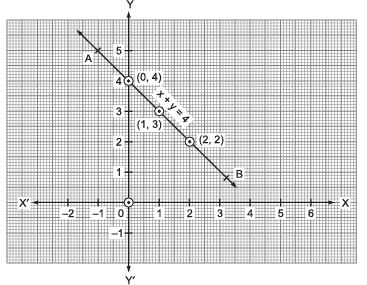Thus, the line AB is the required graph of x + y = 4.

(ii) x – y = 2
⇒ y = x – 2
If we have x = 0,
then y = 0 – 2 = –2 x = 1,
then y = 1 – 2 = – 1 x = 2,
then y = 2 – 2 = 0

∴ We have the following table:

 x 0 1 2 y -2 -1 0

Plot the ordered pairs (0, –2), (1, –1) and (2, 0) on the graph paper. Joining these points, we get a straight line PQ as shown below: Thus, the line PQ is required graph of x – y = 2.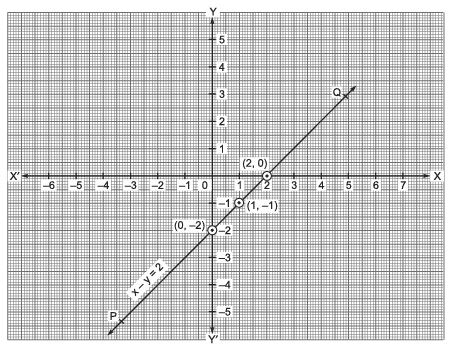(iii) y = 3x

If x = 0, then y = 3(0)
⇒ y = 0 x = 1, then y = 3(1)
⇒ y = 3 x = –1, then y = 3(–1)
⇒ y = –3

∴ We get the following table:

 x 0 1 -1 y 0 3 -3

Plot the ordered pairs (0, 0), (1, 3) and (–1, –3) on the graph paper. Joining these points, we get the straight line LM.
Thus, LM is the required graph of y = 3x.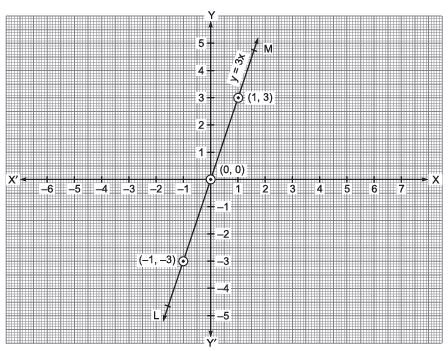Note: The graph of the equation of the form y = kx is a straight line which always passes through the origin.

( iv) 3 = 2x + y
⇒ y = 3 – 2x
∴ If x = 0, then y = 3 – 2(0)
⇒ y = 3 If x = 1, then y = 3 – 2(1)
⇒ y = 1 If x = 2, then y = 3 – (2)
⇒ y = –1

 x 0 1 2 y 3 1 -1

Plot the ordered pairs (0, 3), (1, 1) and (2, –1) on the graph paper. Joining these points, we get a line CD.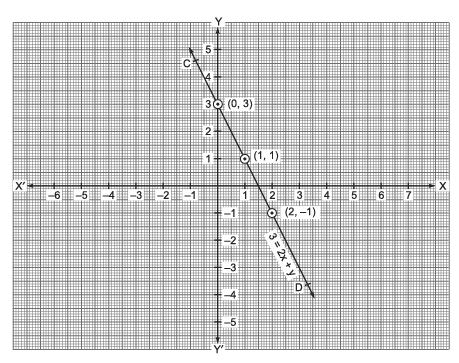Thus, the line CD is the required graph of 3 = 2x + y

Question 2. Give the equations of two lines passing through (2, 14). How many more such lines are there, and why?
Solution: (2, 14) means x = 2 and y = 14
Following equations can have (2, 14) as the solution, i.e. they can pass through the point (2, 14).
(i) x + y = 16

(ii) 7x – y = 0
There can be an unlimited number of lines which can pass through the point (2, 14) because an unlimited number of lines can pass through a point.

Question 3. If the point (3, 4) lies on the graph of the equation 3y = ax + 7, find the value of a.
Solution: The equation of the given line is 3y = ax + 7
∵ (3, 4) lies on the given line.
∴ It must satisfy the equation 3y = ax + 7
We have (3, 4)
⇒ x = 3 and y = 4
L.H.S. = 3y
= 3 x 4
= 12
R.H.S. = ax + 7
= a x 3 + 7
= 3a + 7
∵ L.H.S. = R.H.S.
∴ 12 = 3a + 7
or
3a = 12 – 7 = 5

or
a = (5/3)
Thus, the required value of a is (5/3)

Question 4. The taxi fare in a city is as follows: For the first kilometre, the fare is 8 and for the subsequent distance it is  5 per km. Taking the distance covered as x km and total fare as y, write a linear equation for this information and draw its graph.
Solution: Here, total distance covered = x km
Total taxi fare = ₹  y
Fare for the 1st km = ₹  8
Remaining distance = (x – 1) km
∴ Fare for (x – 1) km = ₹ 5 x (x – 1) km
Total taxi fare = ₹ 8 + ₹  5(x – 1)
∴ According to the condition,
y = 8 + 5(x – 1)
⇒ y = 8 + 5x – 5
⇒ y = 5x + 3
which is the required linear equation representing the given information.
Graph: We have y = 5x + 3
∴ When x = 0, y = 5(0) + 3
⇒ y = 3
When x = –1, y = 5(–1) + 3
⇒ y = –2
When x = –2, y = 5(–2) + 3
⇒ y = –7
∴ We get the following table:

 x 0 -1 -2 y 3 -2 -7

Now, plotting the ordered pairs (0, 3), (–1, –2) and (–2, –7) on a graph paper and joining them, we get a straight line PQ.
Thus, PQ is the required graph of the linear equation y = 5x + 3.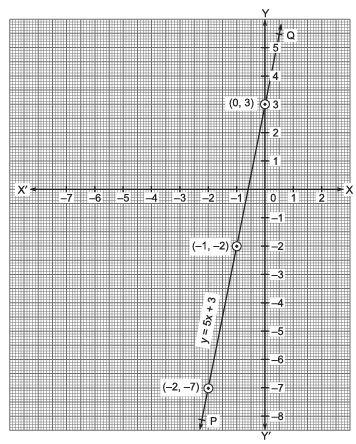Question 5. From the choices given below, choose the equation whose graphs are given in Fig. (i) and Fig.(ii).

 For Fig. (i) For Fig. (ii) (i) y = x (i) y = x + 2 (ii) x + y = 0 (ii) y = x – 2 (iii) y = 2x (iii) y = –x + 2 (iv) 2 + 3y = 7x (iv) x + 2y = 6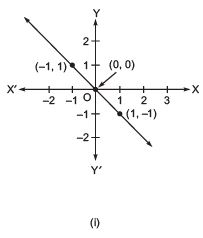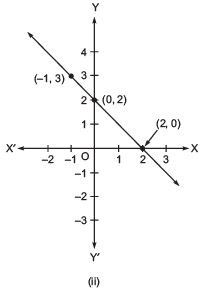Solution: For Fig. (i), the correct linear equation is x + y = 0
[∵ (–1, 1) ⇒ –1 + 1 = 0 and (1, –1)
⇒ 1 + (–1) = 0]
For Fig.

(ii), the correct linear equation is y = –x + 2
[∵ (–1, 3) ⇒ 3 = –(–1) + 2 ⇒ 3 = 3 and (0, 2)
⇒ 2 = –(0) + 2 ⇒ 2 = 2]

Question 6. If the work done by a body on application of a constant force is directly proportional to the distance travelled by the body, express this in the form of an equation in two variables and draw the graph of the same by taking the constant force as 5 units. Also read from the graph the work done when the distance travelled by the body is  (i) 2 units (ii) 0 unit
Solution: Constant force is 5 units.
Let the distance travelled = x units and work done = y units.
Since, Work done = Force x Displacement
⇒ y= 5 x x
⇒ y= 5x
Drawing the graph
We have y = 5x
When x = 0, then y = 5(0) = 0
When x = 1, then y = 5(1) = 5
When x = 1.5, then y = 5(1.5) = 7.5
∴ We get the following table:

 x 0 1 1.5 y 0 5 7.5

Plotting the ordered pairs (0, 0), (1, 5) and (1.5, 7.5) on the graph paper and joining the points, we get a straight line OB.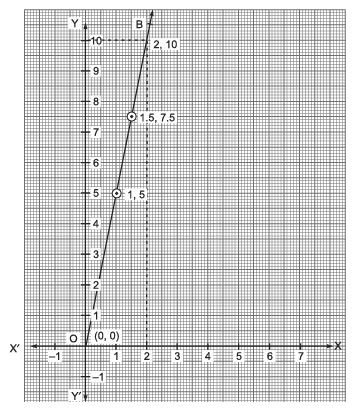From the graph, we get

(i) Distance travelled = 2 units
∴ x = 2, then y = 10 units
⇒ Work done = 10 units.

(ii) Distance travelled = 0 units
∴ y = 5x
⇒ y = 5(0) = 0
∴ Work done = 0 unit.

Question 7. Yamini and Fatima, two students of Class IX of a school, together contributed 100 towards the Prime Minister’s Relief Fund to help the earthquake victims. Write a linear equation which satisfies this data. (You may take their contributions as x and y.) Draw the graph of the same.

Solution: Let the contribution of Yamini = ₹ x and the contribution of Fatima = ₹  y
∴ We have x + y = 100
⇒ y = 100 – x
Now, when x = 0, y = 100 – 0 = 100
When x = 50, y = 100 – 50 = 50
When x = 100, y = 100 – 100 = 0
We get the following table:

 x 0 50 100 y 100 50 0

For drawing the graph, plot the ordered pairs (0, 100), (50, 50) and (100, 0) on a graph paper.
Joining these points we get a line PQ.

Thus, PQ is the required graph of x + y = 100.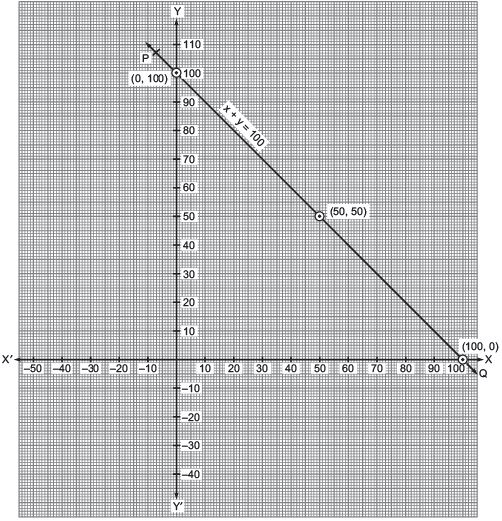Question 8. In countries like USA and Canada, temperature is measured in Fahrenheit, whereas in countries like India, it is measured in Celsius. Here is a linear equation that converts Fahrenheit to Celsius.
F= (9/5)C + 32
(i) Draw the graph of the linear equation above using Celsius for x-axis and Fahrenheit for y-axis.
(ii) If the temperature is 30ºC, what is the temperature in Fahrenheit?
(iii) If the temperature is 95ºF, what is the temperature in Celsius?
(iv) If the temperature is 0ºC, what is the temperature in Fahrenheit and if the temperature is 0ºF, what is the temperature in Celsius?
(v) Is there a temperature which is numerically the same in both Fahrenheit and Celsius? If yes, find it.

Solution: (i) We have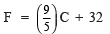When C = 0,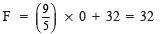When C = –15,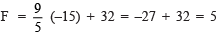When C = –10,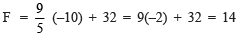We have the following table: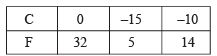Plot the ordered pairs (0, 32), (–15, 5) and (–10, 14) on a graph paper. Joining these points we get a straight line AB.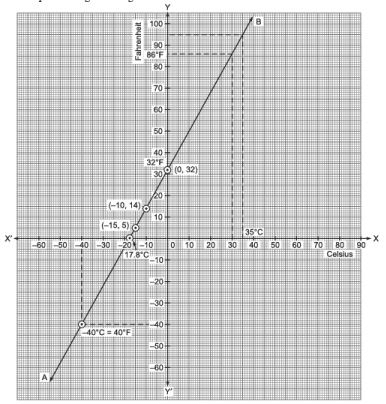(ii) From the graph, we have
86ºF corresponds to 30ºC

(iii) From the graph, we have
95ºF = 35ºC

(iv) From the graph, we have
0ºC = 32ºF
and 0ºF = 17.8ºC

(v) Yes, from the graph, we have
40ºF = –40ºC

## Mathematics (Maths) Class 9

192 videos|230 docs|82 tests

,

,

,

,

,

,

,

,

,

,

,

,

,

,

,

,

,

,

,

,

,

;